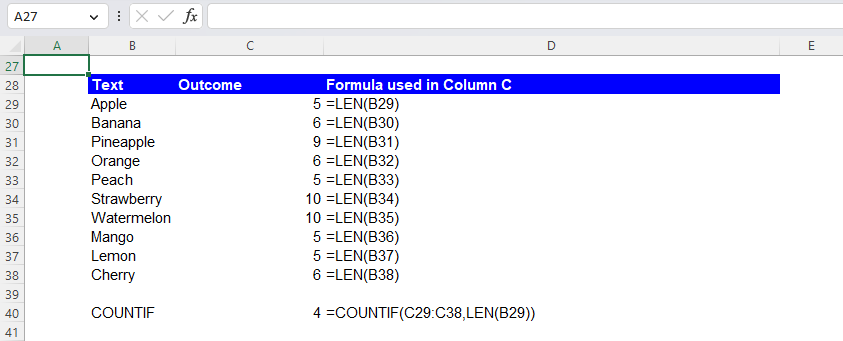Excel Formulas

# LEN Function in Excel: Explained

In this article, you will learn how to use the LEN formula in Excel.

## How does the LEN formula work in Excel?

The LEN formula in Excel returns the number of characters in a given cell. For example, if cell A1 contains the text "Hello World", the formula "=LEN(A1)" would return "11", as there are 11 characters in the text "Hello World".

## When is the LEN function helpful in Excel?

The LEN function in Excel can be helpful in various situations where you need to determine the number of characters in a cell or a range of cells. Examples include:

1. Data validation: You can use the LEN function to ensure that a certain number of characters are entered into a cell. For example, you can use a formula like "=LEN(A1)=5" to check that a cell contains exactly 5 characters.
2. Data cleaning: You can use the LEN function to identify cells that contain a certain number of characters and then use this information to clean your data. For example, you can use the LEN function to identify cells with more than a certain number of characters and then use the TRIM function to remove any extra spaces.

## How do you use the LEN function in Excel?

The general syntax of the LEN formula is as follows:

``=LEN(text)``

text: This argument is mandatory. The number of characters in the selected text string is counted. If a range is chosen as the argument, the LEN function returns the number of characters of text across the same size of the range. For example, if you insert =LEN(A1:A3) in cell B1, where cells A1, A2, and A3 contain X, Y, and Z characters, respectively, the formula gives X, Y, and Z in cells B1, B2, and B3, respectively.

Note: This function may not work correctly for all languages. The formula counts the number of spaces in a text string, including leading and trailing ones.

For example, if you want to count the number of characters in cell B3, you would select the cell where you want to display the result, type "=LEN(B3)", and then press the “Enter” key. The result will be the number of characters in cell B3. The following list shows how many characters each fruit name has.

## What other formulas can the LEN function be used together in Excel?

For example, you can use the LEN formula with the IF function as below.

``=IF(LEN(B16)>5, "Too many characters", "OK")``

This combined formula checks if the number of characters in cell B16 is greater than 5. If it is, the function returns "Too many characters". Otherwise, it returns "OK". As an example, we inserted this combined formula from C16 to C25 to test items in the range of B16:B25 as below.

Another example is the COUNTIF function. You can use the LEN function to count the characters in a cell and then use the COUNTIF function to count the number of cells with the same number of characters. For example, you can use a formula like the one below.

``=COUNTIF(C29:C38, LEN(B29))``

This formula counts the number of cells in the range C29:C38 that have the same number of characters as cell B29, as shown in the picture below.How to use the LEN function with the COUNTIF formula in Excel

The last example is the TRIM function. You can use the LEN function to identify cells that contain extra spaces, then use the TRIM function to remove those spaces. For example, you can insert a formula to check if there are extra spaces in cell B44 as follows.

``=IF(LEN(B44)>LEN(TRIM(B44)), "Extra spaces", "No extra spaces")``

All items in the range of B44:B48, except for A44, contain extra spaces, so the combined formula returns “Extra spaces” in the corresponding outcome cells.

## Analyze your live financial data in a snap in Google Sheets

Are you learning this formula to visualize financial data, build a financial model, or conduct financial analysis? In that case, LiveFlow may help you automate manual workflows, update numbers in real-time, and save time. You can access various financial templates on our website, from the simple Income Statement to Multi-Currency Consolidated Financial Statement. Are you interested in this product but are an Excel user? That’s not a problem at all. You can connect Google Sheets to Excel quickly.